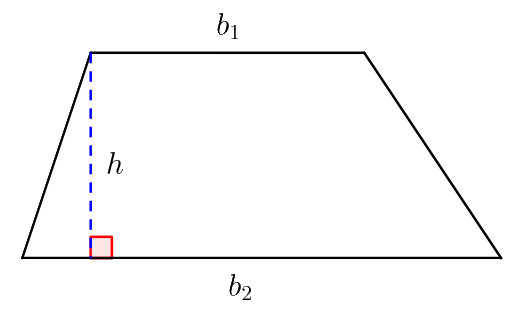# URI - BEECROWD - BEE 1043 | Triangle Solution in C,C++,Python | URI - BEECROWD - BEE 1043 Solution

## URI - BEECROWD - BEE Online Judge Solution  1043 | Triangle - URI - BEECROWD - BEE 1043 Solution in C,C++,Python

Read three point floating values (A, B and C) and verify if is possible to make a triangle with them. If it is possible, calculate the perimeter of the triangle and print the message:

Perimetro = XX.X

If it is not possible, calculate the area of the trapezium which basis A and B and C as height, and print the message:

Area = XX.X

## Input

The input file has tree floating point numbers.

## Output

Print the result with one digit after the decimal point.

 Input Sample Output Sample 6.0 4.0 2.0 Area = 10.0
 6.0 4.0 2.1 Perimetro = 12.1

### URI Online Judge Solution  1043 | Triangle - URI 1043 Solution in C,C++,Python:

Demonstration:

How it works?
Check if it is possible to make a triangle with three integer numbers. We know that the sum of two sides is greater than the third side of a triangle.Here we should check this exactly.Next the perimeter, It is the sum of all three sides. Hopefully you would understand the problem.

N.B: Don't copy paste the code as same. Just try to understand it and try yourself. It would be better for you.

### What is the Perimeter of a Triangle?

The sum of the lengths of the sides is the perimeter of any polygon. In the case of a triangle,

Perimeter = Sum of the three sides

### Perimeter of Triangle Formula:

The formula for the perimeter of a closed shape figure is usually equal to the length of the outer line of the figure. Therefore, in the case of a triangle, the perimeter will be the sum of all the three sides. If a triangle has three sides a, b and c, then,

Perimeter, P = a + b +c

Area of a Trapezium:

trapezium, also known as a trapezoid, is a quadrilateral in which a pair of sides are parallel, but the other pair of opposite sides are non-parallel. The area of a trapezium is computed with the following formula:

\text{Area}=\frac {1}{2} × \text {Sum of parallel sides} × \text{Distance between them}.The parallel sides are called the bases of the trapezium. Let $b_1$ and $b_2$ be the lengths of these bases. The distance between the bases is called the height of the trapezium. Let $h$ be this height. Then this formula becomes:

\text{Area}=\frac{1}{2}(b_1+b_2)h

URI Problem 1043 Solution in C :

 URI Online Judge 1043 Solve  in C :
#include<stdio.h>
int main()
{
float a,b,c,perimeter,area;
scanf("%f%f%f",&a,&b,&c);

if((a+b)>c&&(b+c)>a&&(c+a)>b){
perimeter=a+b+c;
printf("Perimetro = %.1f\n",perimeter);
}
else{
area=.5*(a+b)*c;
printf("Area = %.1f\n",area);
}
return 0;
}